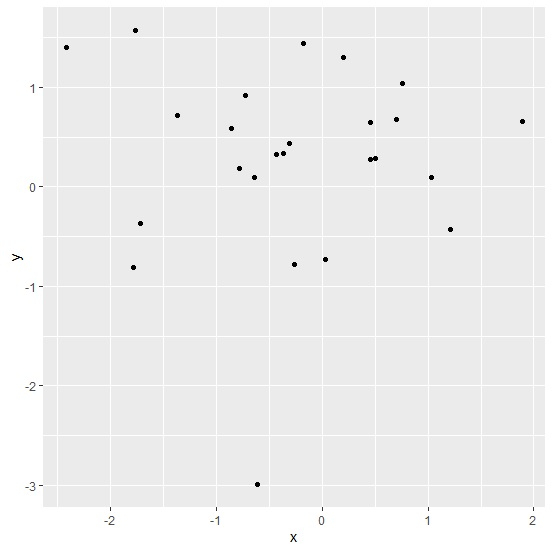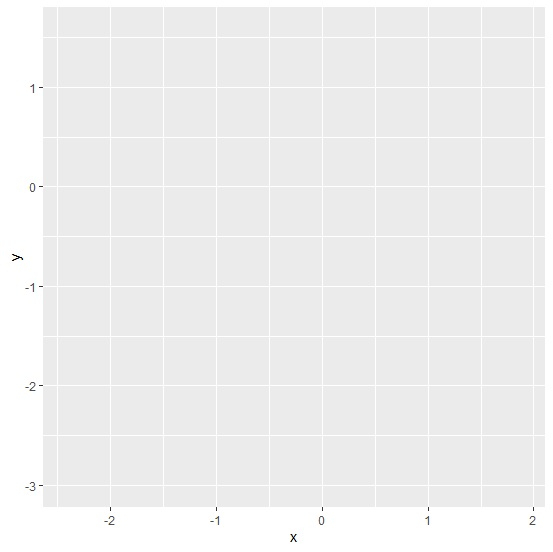# How to create a ggplot2 graph in R without showing values?

To create a ggplot2 graph in R without showing values, we can follow the below steps −

• First of all, create a data frame.
• Then, create the graph using ggplot2.
• Create the graph using ggplot2 without providing the function for the plot.

## Create the data frame

Let's create a data frame as shown below −

Live Demo

x<-rnorm(25)
y<-rnorm(25)
df<-data.frame(x,y)
df

On executing, the above script generates the below output(this output will vary on your system due to randomization) −

        x          y
1 -0.30663859 0.43281803
2 -1.78130843 -0.81139318
3 -0.17191736 1.44410126
4 1.21467470 -0.43144620
5 1.89519346 0.65564788
6 -0.43046913 0.32192527
7 -0.25726938 -0.78383894
8 -1.76316309 1.57572752
9 0.46009735 0.64289931
10 -0.63999488 0.08976065
11 0.45545012 0.27655075
12 0.70483734 0.67928882
13 1.03510352 0.08983289
14 -0.60892638 -2.99309008
15 0.50495512 0.28488295
16 -1.71700868 -0.36723464
17 -0.78445901 0.18523056
18 -0.85090759 0.58182373
19 -2.41420765 1.39973683
20 0.03612261 -0.72729206
21 0.20599860 1.30254263
22 -0.36105730 0.33584812
23 0.75816324 1.03850610
24 -0.72670483 0.92072857
25 -1.36828104 0.72087816

## Create the graph using ggplot2

Use geom_point function to create scatterplot between x and y −

x<-rnorm(25)
y<-rnorm(25)
df<-data.frame(x,y)
library(ggplot2)
ggplot(df,aes(x,y))+geom_point()

### Output## Create the graph using ggplot2 without plot function

Use ggplot function to create scatterplot between x and y but do not give plotting function geom_point −

x<-rnorm(25)
y<-rnorm(25)
df<-data.frame(x,y)
library(ggplot2)
ggplot(df,aes(x,y))

### Output